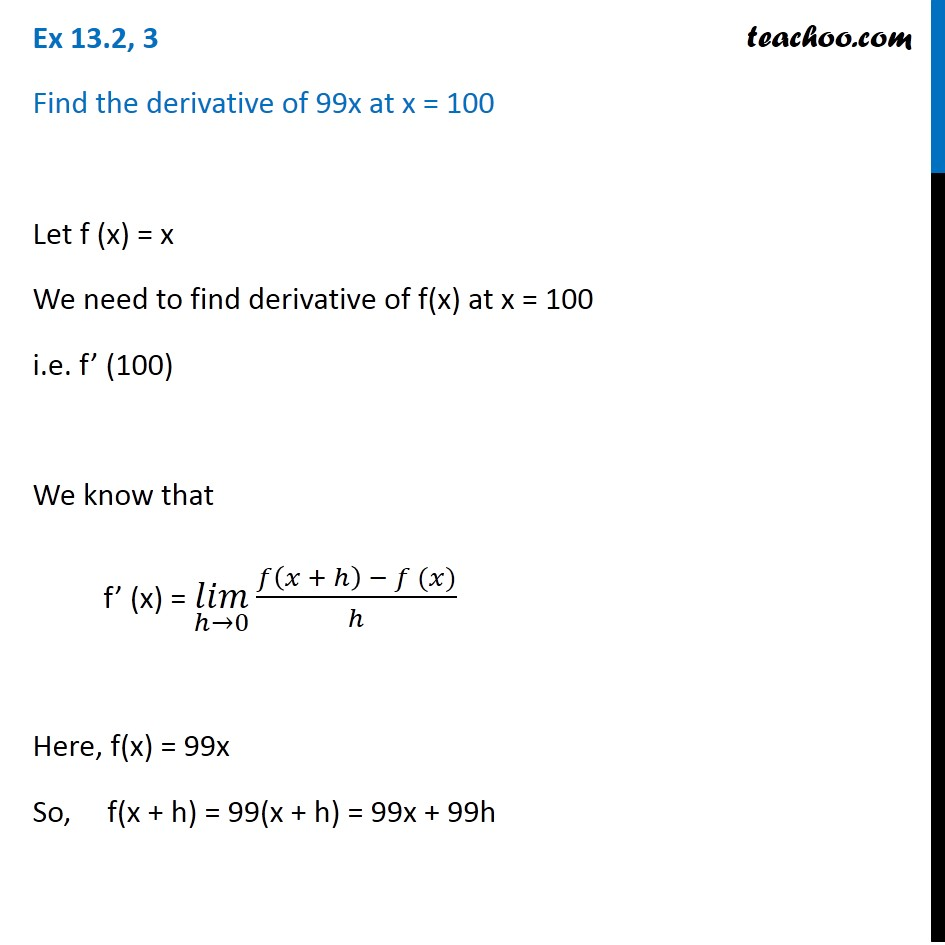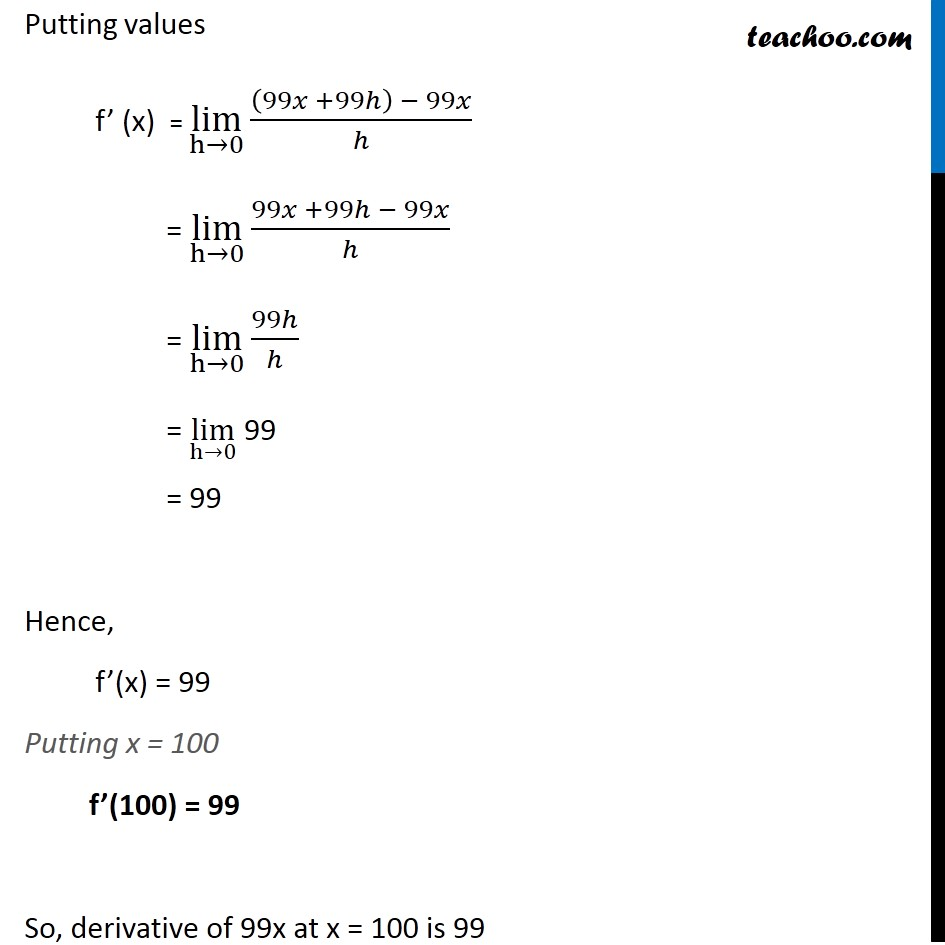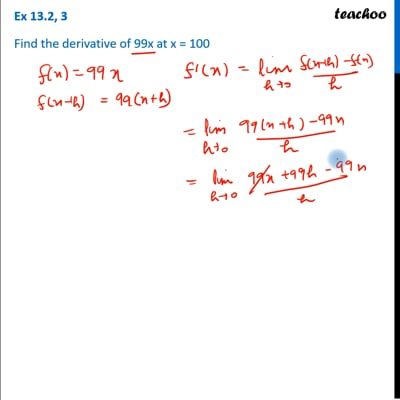Ex 12.2

Chapter 12 Class 11 Limits and Derivatives
Serial order wiseThis video is only available for Teachoo black users

Learn in your speed, with individual attention - Teachoo Maths 1-on-1 Class

### Transcript

Ex 12.2, 3 Find the derivative of 99x at x = 100 Let f (x) = x We need to find derivative of f(x) at x = 100 i.e. f’ (100) We know that f’ (x) = (𝑙𝑖𝑚)┬(ℎ→0)⁡〖(𝑓(𝑥 + ℎ) − 𝑓 (𝑥))/ℎ〗 Here, f(x) = 99x So, f(x + h) = 99(x + h) = 99x + 99h Putting values f’ (x) = lim┬(h→0)⁡〖((99𝑥 +99ℎ) − 99𝑥)/ℎ〗 = lim┬(h→0)⁡〖(99𝑥 +99ℎ − 99𝑥)/ℎ〗 = lim┬(h→0)⁡〖99ℎ/ℎ〗 = lim┬(h→0) 99 = 99 Hence, f’(x) = 99 Putting x = 100 f’(100) = 99 So, derivative of 99x at x = 100 is 99